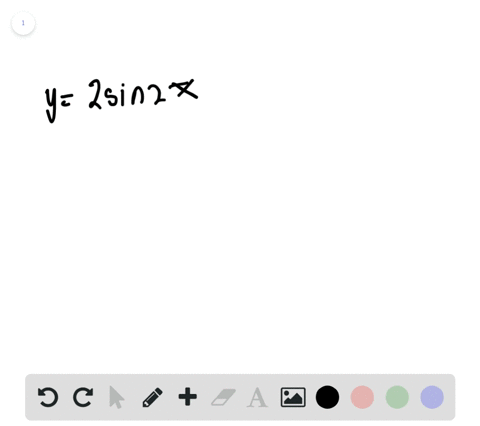🎉 The Study-to-Win Winning Ticket number has been announced! Go to your Tickets dashboard to see if you won! 🎉View Winning Ticket### Period and Amplitude In Exercises $45-48,$ determ…

00:41University of Southern California
Problem 44

# Height of a Mountain While traveling across flat land, you notice a mountain directly in front of you. Its angle of elevation (to the peak) is $3.5^{\circ}$ . After you drive 13 miles closer to the mountain, the angle of elevation is $9^{\circ}$ . Approximate the height of the mountain.

## Discussion

You must be signed in to discuss.

## Video Transcript

Theo word problem is as follows. While traveling across flat land, you notice a mountain directly in front of you. It's angle of elevation to the peak is 3.5 degrees. After you, Dr. 13 miles closer to the mountain. The angle of elevation is now nine degrees. Approximate the height of the mountain. All right, so you see, we have this diagram right here of the word problem, and it shows how, after moving 13 miles, the angle of elevation changes. And so to begin, we want to find ways that we can incorporate trigonometry and defining this problem. And the easiest way to do that would we by creating a right triangle in our diagram. So I'm just gonna draw this vertical line and add that right triangle symbol. So we know it's a right triangle. Now, we should go about labeling our diagram to identify what we want to solve than any other pieces of information we may need, Um, while looking for that height. So I'm going to label the height of the mountain age just so we know what to solve for and this little bit of distance right here from our nine degree angle of elevation to the vertical of that man. I'm going to call that eggs and then just for simplicity. I'm going to give each of these angle values a symbol. And so 3.5 degrees is going to be equal to theta, and nine degrees 3.5 degrees is equal to fei and then nine degrees of equal to data. So to begin, we should start by taking account of any Trigon in metric relationships we see. So if we look it, fi, we see that Tangent Fi is equivalent to H over 13 point X. That's that opposite over adjacent. And then we see that tangent data his equivalent to H over eggs. No, in this case, we have to equations, um, two unknowns, and that's enough to allow us to sell for age. So let's begin doing our algebra toe, isolate H and then solve for him. So what I'll do is I want to substitute um h from this equation or I want to substitute out X and this one. So what we'll do is we'll solve for X over here, and we can say that X equals h divided by tangent Fada and they were going to bring that over here in seven. We'll have tangent if I because h over 13 plus now we're going to serve that in a church over tangent, Fada. Now I'm going to multiply each side by the denominator on your right. So I have tangent fi times 13 plus each over tangent. Seita equals H. And then I'm going to distribute this tangent data. I'm follow this arrow and bring it up here. We'll have 13 tangent. Oh, fi plus tangent of fi over Tangent of data H equals H. Now I'm going to subtract this H term to the other side just so that we can isolate age. We'll have 13 tangent of Fei equals h minus tan by over tan they th factor out on H one minus tan Bye over 10 data equals 13 10 5 And then I'm just going to divide both sides by the quantity contained within these parentheses and we'll see that 13 10 5 divided by one minus 10 5 divided by 10 Data equals H. So if you guys use your calculator and plug in our values of data and fine, you'll see that the approximate value of H is one point 295 miles. That's a pretty tall melon